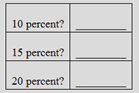# Answer the following questions. a. If a bank had reserves of $30,000 and demand deposits of$200,000 (and no other deposits), how much could it lend out if faced a required reserve ratio of b. If the bank then received a new $40,000 deposit in a customer's demand deposit account, how much could it now lend out if it faced a required reserve ratio of ÂBuyFindarrow_forward ### Exploring Economics 8th Edition Robert L. Sexton Publisher: SAGE Publications, Inc ISBN: 9781544336329 #### Solutions Chapter SectionBuyFindarrow_forward ### Exploring Economics 8th Edition Robert L. Sexton Publisher: SAGE Publications, Inc ISBN: 9781544336329 Chapter 25, Problem 16P Textbook Problem 3 views ## Answer the following questions.a. If a bank had reserves of$30,000 and demand deposits of $200,000 (and no other deposits), how much could it lend out if faced a required reserve ratio ofb. If the bank then received a new$40,000 deposit in a customer's demand deposit account, how much could it now lend out if it faced a required reserve ratio ofÂTo determine

(a)

To compute:

The amount of money the bank could lend if a bank had a reserve of $30,000 and demand deposits of$200,000.

### Explanation of Solution

Given information:

The bank had reservesof $30,000 and demand deposits of$200,000. The bank reserve ratio is 10%.

Calculation of required reserve:

Requiredreserve=r×D=0.1×$200,000=$20,000

Calculation of excess reserve:

Excessreserve=ActualreserveRequiredreserve=$30,000$20,000=$10,000 The assets and liability of the bank should be equal. Therefore, Demanddepoists+Excessreserve=Reserve+Loans$200,000+$10,000=$30,000+LoansLoans=$210,000$30,000=$180,000 Similarly, The bank had reservesof$30,000 and demand deposits of $200,000. The bank reserve ratio is 15%. Calculation of required reserve: Requiredreserve=r×D=0.15×$200,000=$30,000 Calculation of excess reserve: Excessreserve=ActualreserveRequiredreserve=$30,000$30,000=$0

The assets and liability of the bank should be equal

To determine

(b)

To compute:

The additional dollar that can be lent out as a result of \$40,000 deposit.

### Still sussing out bartleby?

Check out a sample textbook solution.

See a sample solution

#### The Solution to Your Study Problems

Bartleby provides explanations to thousands of textbook problems written by our experts, many with advanced degrees!

Get Started

Find more solutions based on key concepts
Why is productivity important?

Principles of Microeconomics (MindTap Course List)

Indicate using a (+), (), or (0) whether each of the following events would probably cause accounts receivable ...

Fundamentals of Financial Management, Concise Edition (with Thomson ONE - Business School Edition, 1 term (6 months) Printed Access Card) (MindTap Course List)

Should the United States attempt to reduce air and water pollution to zero? Why or why not?

Microeconomics: Private and Public Choice (MindTap Course List)